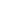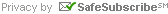About Us | Stations for Sale | Active Buyers | Station Appraisals | Helpful Resources Newsletter | Radio Station References | Site Map | Contact UsTweetSign up for our Email NewsletterFor Email Marketing you can trust

# Helpful Resources >>  Converting Channel Numbers to Frequencies

« Back to Helpful Resources main

 201 = 88.1 226 = 93.1 251 = 98.1 276 = 103.1 202 = 88.3 227 = 93.3 252 = 98.3 277 = 103.3 203 = 88.5 228 = 93.5 253 = 98.5 278 = 103.5 204 = 88.7 229 = 93.7 254 = 98.7 279 = 103.7 205 = 88.9 230 = 93.9 255 = 98.9 280 = 103.9 206 = 89.1 231 = 94.1 256 = 99.1 281 = 104.1 207 = 89.3 232 = 94.3 257 = 99.3 282 = 104.3 208 = 89.5 233 = 94.5 258 = 99.5 283 = 104.5 209 = 89.7 234 = 94.7 259 = 99.7 284 = 104.7 210 = 89.9 235 = 94.9 260 = 99.9 285 = 104.9 211 = 90.1 236 = 95.1 261 = 100.1 286 = 105.1 212 = 90.3 237 = 95.3 262 = 100.3 287 = 105.3 213 = 90.5 238 = 95.5 263 = 100.5 288 = 105.5 214 = 90.7 239 = 95.7 264 = 100.7 289 = 105.7 215 = 90.9 240 = 95.9 265 = 100.9 290 = 105.9 216 = 91.1 241 = 96.1 266 = 101.1 291 = 106.1 217 = 91.3 242 = 96.3 267 = 101.3 292 = 106.3 218 = 91.5 243 = 96.5 268 = 101.5 293 = 106.5 219 = 91.7 244 = 96.7 269 = 101.7 294 = 106.7 220 = 91.9 245 = 96.9 270 = 101.9 295 = 106.9 221 = 92.1 246 = 97.1 271 = 102.1 296 = 107.1 222 = 92.3 247 = 97.3 272 = 102.3 297 = 107.3 223 = 92.5 248 = 97.5 273 = 102.5 298 = 107.5 224 = 92.7 249 = 97.7 274 = 102.7 299 = 107.7 225 = 92.9 250 = 97.9 275 = 102.9 300 = 107.9